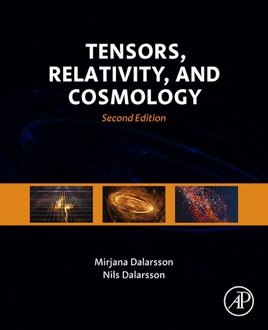• \$144.99

## Publisher Description

Tensors, Relativity, and Cosmology, Second Edition, combines relativity, astrophysics, and cosmology in a single volume, providing a simplified introduction to each subject that is followed by detailed mathematical derivations.

The book includes a section on general relativity that gives the case for a curved space-time, presents the mathematical background (tensor calculus, Riemannian geometry), discusses the Einstein equation and its solutions (including black holes and Penrose processes), and considers the energy-momentum tensor for various solutions. In addition, a section on relativistic astrophysics discusses stellar contraction and collapse, neutron stars and their equations of state, black holes, and accretion onto collapsed objects, with a final section on cosmology discussing cosmological models, observational tests, and scenarios for the early universe.

This fully revised and updated second edition includes new material on relativistic effects, such as the behavior of clocks and measuring rods in motion, relativistic addition of velocities, and the twin paradox, as well as new material on gravitational waves, amongst other topics.
Clearly combines relativity, astrophysics, and cosmology in a single volumeExtensive introductions to each section are followed by relevant examples and numerous exercisesPresents topics of interest to those researching and studying tensor calculus, the theory of relativity, gravitation, cosmology, quantum cosmology, Robertson-Walker Metrics, curvature tensors, kinematics, black holes, and moreFully revised and updated with 80 pages of new material on relativistic effects, such as relativity of simultaneity and relativity of the concept of distance, amongst other topicsProvides an easy-to-understand approach to this advanced field of mathematics and modern physics by providing highly detailed derivations of all equations and results